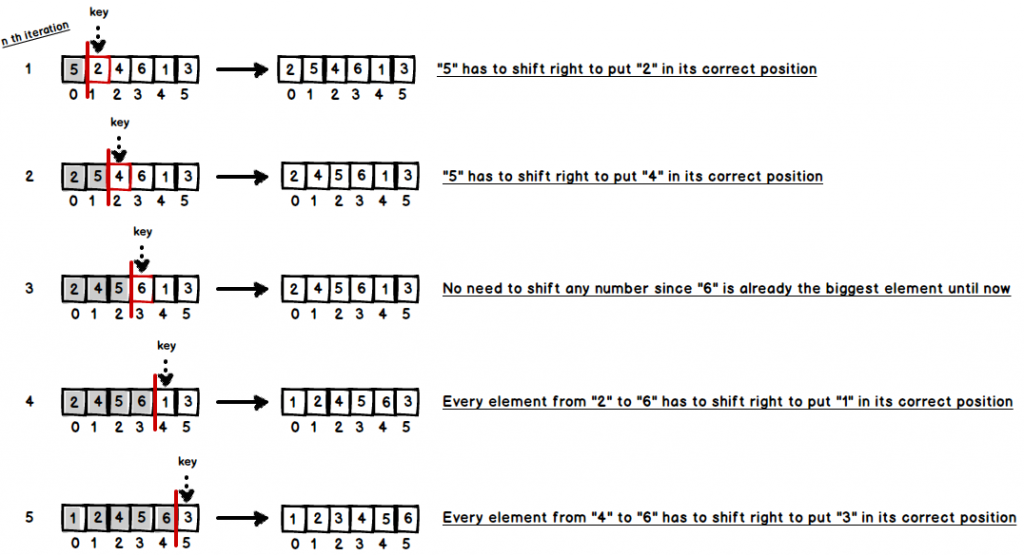# Insertion Sort

Insertion sort is an efficient algorithm for sorting a small number of elements, however, it is less efficient on large lists than more advanced sorting algorithms. On the other hand, it has several advantages which are;

• It is adaptive; Sorting performance adapts to the initial order of elements.
• It is online; New elements can be added during the sorting phase.
• It is stable; The elements with equal key will keep their order in the array.
• It is in-place; Requires constant amount of space $$O(1)$$.

## Algorithm

The main idea of insertion sort is that array is divided in two parts which left part is already sorted, and right part is unsorted. So, at every iteration sorted part grows by one element which is called key. During an iteration, if compared element is greater than key then compared element has to shift to right to open a position for key. Lets see an iterative example on array {5, 2, 4, 6, 1, 3}. In each turn the key is underlined, and the sorted part of array has bold numbers.## Complexity Analysis

Time Space
Best case Worst case Average case Worst case
$$O(n)$$ $$O(n^{2})$$ $$O(n^{2})$$ $$O(1) auxiliary$$

## Code

public class InsertionSort {

public void insertionSort(int[] numbers) {
for (int j = 1; j < numbers.length; j++) {
int key = numbers[j];
int i = j - 1;

while (i >= 0 && numbers[i] > key) {
numbers[i + 1] = numbers[i];
i = i - 1;
}

numbers[i + 1] = key;
}
}
}


And here are a bunch of test cases.

public class InsertionSortTest {
private InsertionSort testClass;

@Before
public void setUp() {
testClass = new InsertionSort();
}

@Test
public void insertionSortEx1TestSuccess() throws Exception {
int[] numbers = new int[] { 9, 8, 7, 6, 5, 4, 3, 2, 1 };
testClass.insertionSort(numbers);

assertArrayEquals(new int[] { 1, 2, 3, 4, 5, 6, 7, 8, 9 }, numbers);
}

@Test
public void insertionSortEx2TestSuccess() throws Exception {
int[] numbers = new int[] { 5, 2, 4, 6, 1, 3 };
testClass.insertionSort(numbers);

assertArrayEquals(new int[] { 1, 2, 3, 4, 5, 6 }, numbers);
}
}